Engineering Jobs   »   Mechincal Engineering quizs   »   UPPSC Lecturer Recruitment 2021, UPPSC Lecturer...

# UPPSC Lec’21 ME: Daily Practices Quiz. 25-Nov-2021

Quiz: Mechanical Engineering
Exam: UPPSC AE/Lecturer
Topic: Industrial

Each question carries 3 marks
Negative marking: 1 mark
Time: 8 Minutes

Q1. At EOQ, if the ordering cost is Rs. 500 and the shortage cost is Rs. 100 then what will be the holding cost?
(a) Rs. 5
(b) Rs. 600
(c) Rs. 400
(d) Rs. 10

Q2. In an inventory, if there is production and shortage both are involved, then what is the correct formula of EOQ? Where D be the annual demand C_o be the ordering cost, C_h be the holding cost, p be the p be the rate of production, d be the demand or consumption rate and C_b be the shortage cost.
(a) √((2DC_o)/C_h ×p/(p-d)×(C_b+C_h)/C_b )
(b) √((2DC_o)/C_h ×p/(p-d)×(C_b-C_h)/C_b )
(c) √((2DC_o)/C_h ×(p-d)/p×(C_b+C_h)/C_b )
(d) √((2DC_o)/C_h ×p/(p+d)×(C_b+C_h)/C_b )

Q3. What is the reorder level when its lead time is 7 days, consumption rate of a quantity is 20 per day and its safety stock is 50?
(a) 370
(b) 200
(c) 150
(d) 190

Q4. If the cost of goods sold is Rs. 200 and the cost of average inventory is Rs. 150 then the value of inventory turn-over ratio is?
(a) 9/16
(b) 4/3
(c) 3/4
(d) 16/9

Q5. The rules for the construction of network in PERT and CPM are
1. No two or more activities may have the same head and tail event
2. Dummy activity only be used when it is necessary but there is some restriction on the number of dummy activities used
3. There should be no LOOPING and DANGLING on the network diagram
Which of the following is correct about above statement?
(a) Only 1 and 3
(b) Only 1 and 2
(c) Only 2 and 3
(d) All are correct

Q6. The FULKERSON’S Rule is used
(a) To numbering the event in the network diagram
(b) To arrange the event according to zero slack
(c) To calculate total slack time
(d) To calculate total number of dummy activities used in the network

Solutions

S1. Ans. (c)
Sol. As we know that, at EOQ
Ordering cost=Holding cost+Shortage cost
500=Holding cost+100
Holding cost=500-100=Rs.400
S2. Ans. (a)
Sol. If both production and shortage case is considered in an Inventory then the formula of EOQ is
Q^*=√((2DC_o)/C_h ×p/(p-d)×(C_b+C_h)/C_b )
And its total inventory cost (TIC) at EOQ is
〖TIC〗^*=√(2DC_o C_h×p/(p-d)×(C_b+C_h)/C_b )
S3. Ans. (d)
Sol. We know that, ROL=LT×d+SS
Where ROL = Reorder level, LT = Lead time = 7 days, d = consumption rate = 20 and SS = safety stock = 50
ROL=7×20+50=140+50=190

S4. Ans. (b)
Sol. Inventory turn-over ratio (ITR)
ITR=(Cost of goods sold)/(Cost of average inventory)=200/150=4/3

S5. Ans. (a)
Sol. The rules for the construction of network in PERT and CPM are
1. No two or more activities may have the same head and tail event
2. Dummy activity only be used when it is necessary but there is no restriction on the number of dummy activities used
3. There should be no LOOPING and DANGLING on the network diagram
4. The length and direction of arrow is indicative and the time flow from left to right on network diagram
5.An activity ca only be started when all the activities earlier to it are finished.

S5. Ans. (a)
Sol. The FULKERSON’S Rule is used to numbering the event in the network diagram. When the network diagram is complex and it seems difficult to number the event then The FULKERSON’S Rule is used.

Sharing is caring!

•GATE 2023 Admit Card Out, Direct Link fo...
•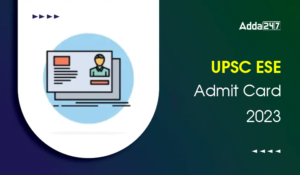UPSC ESE Admit Card 2023, Download Preli...
•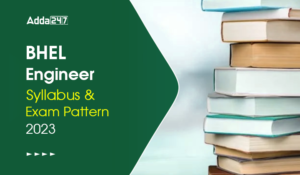BHEL Engineer Trainee Syllabus and Exam ...
•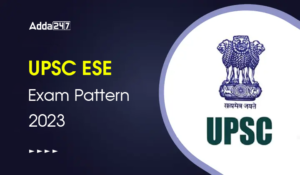UPSC ESE Exam Pattern 2023, Check UPSC E...
•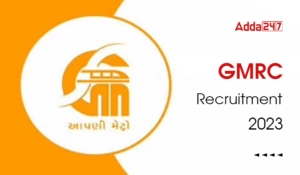GMRC Recruitment 2023, Apply Online For ...
•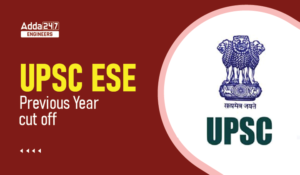UPSC ESE Previous Year Cutoff, Check Yea...
•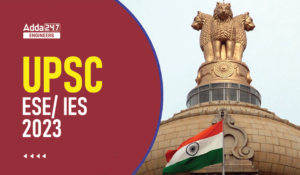UPSC ESE/IES 2023 Notification, Apply On...